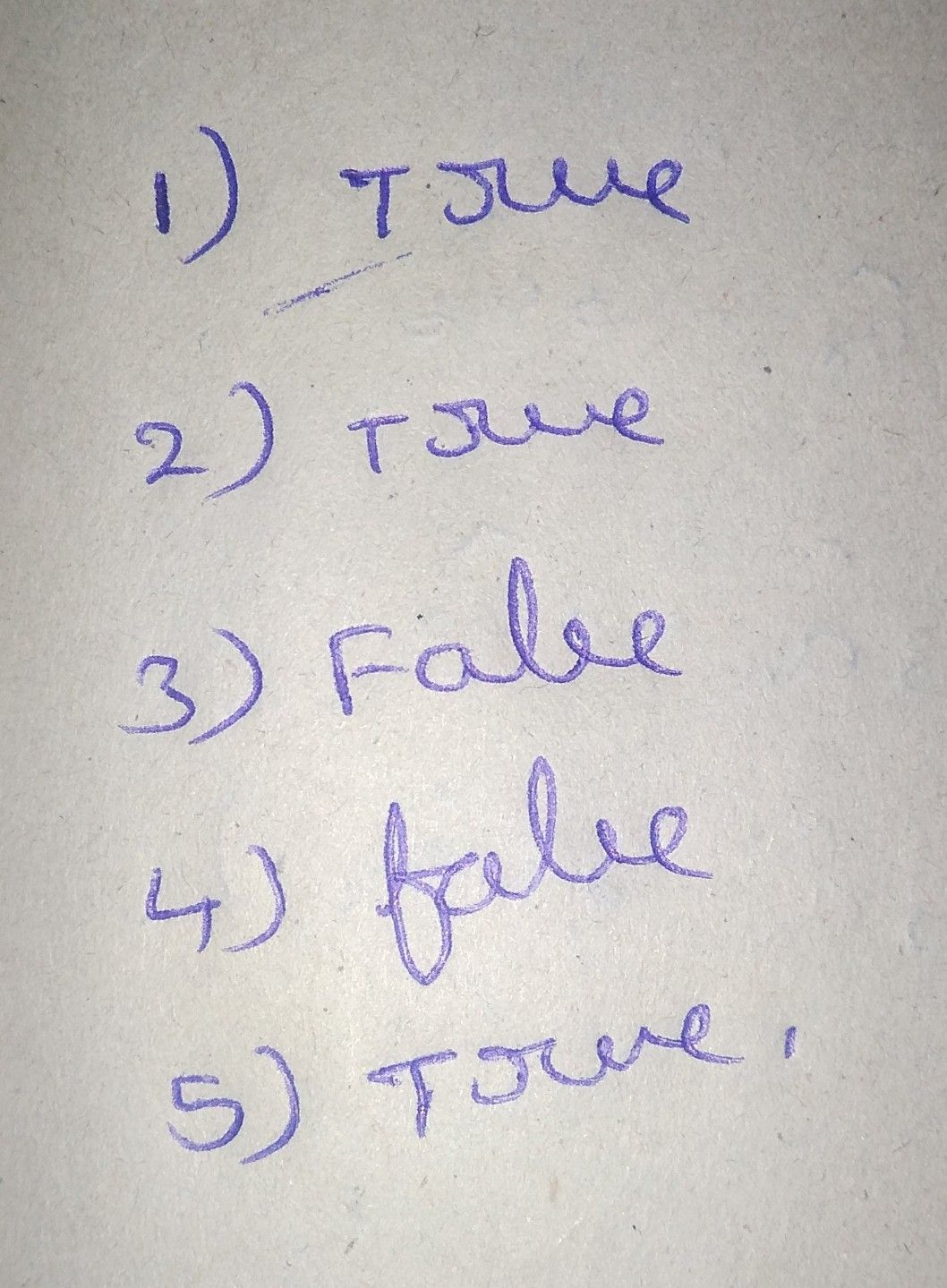Symbol
Problem$A$ Based on your understanding of the lesson, complete the following sentences $1.A$ real number has a that can $oQ$ described as Rational Irrational. 2. The square $o1a$ Rational Number is considered 3. When a number is Perfect Square, then its Principal Root is 4. When the Principal Root of a number is non-terminating or $non-rcpcating$ decima then that Principal Root is described as 5. When extracting the Principal Root of a real number, it can be described as either or B. Refer to the statements below and tell whether the given statement is TRUE or FALSE. Write your answer on the space provided. 1. Estimating is one way to determine where the irrational square root $ofa$ number lie. 2. The square root of $25isa$ perfect square. 3. $Tha$ square root of $30$ lies between 3 and 4. $4$ $Thc$ square root of $121$ lies between $10a$ and $11$ $5$ Only perfect square integers have a square $r0ot$ $5$
7th-9th grade
Algebra
Search count: 125
SolutionQanda teacher - Aravind